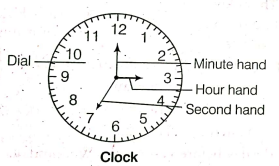## Clocks

### clocks

CLOCK:

A clock is a circular/square shaped instrument used to view/measure time in hours, minutes and seconds.
There are mainly four important parts of a clock-dial, hour hand, minute hand and second' hand.

The face or dial of a watch is a circle whose circumference is divided into 60 equal parts, called minute spaces.

A clock has two hands, the smaller one is called the hour hand or short-hand while the larger one is called the minute hand or long band.$$60\ seconds =1 \ minute$$

$$60 \min =1 \ hour$$

$$30 \ min = Half \ of \ an \ hour$$

$$15 \ min = Quarter \ of \ an\ hour$$

### important points to remember:

1. In 60 minutes, the minute hand gains 55 minutes on the hour hand.
2. In every hour, both the hands coincide once.
3. The hands are in the same straight line when they are coincident or opposite to each other.
4. When the two hands are at right angles, they are 15-minute spaces apart.
5. When the hands are in opposite directions, they are 30-minute spaces apart.
6. Angle traced by hour hand in 12 hrs =\$$360^{\circ}$$.
7. Angle traced by minute hand in 60 min. =$$360^{\circ}$$.
8. Too Fast and Too Slow: If a watch or a clock indicates 8.15, when the correct time is 8, it is said to be 15 minutes too fast.
9. On the other hand, if it indicates 7.45 when the correct time is 8, it is said to be It minutes too slow.

### key points

• Both hands of a clock occur at a right angle twice in one hour, 22 times in 12 h and 44 times in 24 h.
• Both hands of a clock occur in a straight line or are opposite once in one hour, 11 times in 12 h and 22 times in 24 h
• The hands of a clock coincide once in an hour, 11 times in 12 h and 22 times in 24 h.